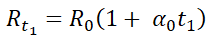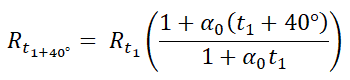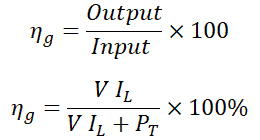Electrical Technology

# Swinburne’s Test of DC Machines

## Swinburne’s Test of DC Motors & Generators – Circuit, Calculation, Advantages & Applications

### What is Swinburne’s Test?

Swinburne’s test (named after James Swinburne) is an indirect method of testing a DC shunt or DC compound motor. During this test, the motor is not loaded. Therefore, this method of testing is also known as No-load Test. The Swinburne’s test is very helpful for very large machines that cannot be tested on actual load. So, this method helps to determine the performance characteristics of large DC machines.

The Swinburne’s test is performed to calculate the losses and efficiency of the motor at any desired load. In this test, the DC machine operates as a motor running on no load. The excitation is given to the motor to operate on rated voltage and rated speed. The connection diagram of the Swinburne’s test is shown in the figure below.

### Circuit DiagramPerformance

The connection diagram of the Swinburne’s test for the DC shunt motor is shown in the above figure. Here,

• V = Supply Voltage
• ISh = Shunt field Current

In no-load condition, the current flows through the armature are;

Ia0 = I0ISh

No-load Input power = V I0

The no-load power is used to supply;

• Iron loss in the core
• Friction loss in commutator and bearings
• Windage loss
• Armature copper loss at no-load

When the machine is loaded, the temperature of the field winding and armature winding is increased. During this test, the resistance of both windings is measured using the voltmeter-ammeter method. And the temperature of a winding is at room temperature (let’s say ). Consider, when the machine is loaded, the temperature rise of winding is approximately 40-50˚ C.

So, we need to add a hot resistance to avoid temperature rise. The hot resistance allows a temperature rise of 40˚ C and the calculation is as follows;For 40˚ C;Where, α0 = temperature coefficient of resistance at 0˚ C.Now, the supply voltage (V) is measured by voltmeter V, motor input current (I0) is measured by ammeter A1, and the shunt field current (Ish) is measured by ammeter A2.

Here, the DC machine operates on a no-load. Hence, we can consider the mechanical loss as zero. And the input power is used to supply internal losses in a machine that includes; Shunt field copper loss, Armature copper loss, and Stray power loss in a shunt wound machine. In the case of a compound wound machine, it also includes series field copper loss.

Input power at no-load = V I0 Watt

Shunt field copper loss = V ISh Watt

From the circuit diagram, the current passes through the armature Ia in the no-load condition is;

Ia0 = I0ISh

So, the armature copper loss = I2a0 Ra = (I0ISh)2 Ra (where Ra = hot resistance of armature)

Stray power loss, Ps = Input on no-load – Shunt field loss – armature copper loss

Ps = V I0V ISh (I0 – ISh)2 Ra

Constant losses, Pc = Ps + Shunt field copper loss

PC = V I0V ISh (I0 – ISh)2 Ra + V ISh

PC = V I0(I0 – ISh)2 Ra

### Efficiency Calculation

#### Efficiency of DC Machine When it is Running as a Motor

Assume;

Total input power = V ILWatts

Total constant losses are the same as determined above;

Total constant losses = PC = V I0(I0 – ISh)2 Ra

Armature copper loss = (I0 – ISh)2 Ra

Total losses = PT = PC(I0 – ISh)2 Ra = V I0 – (I0 – ISh)2 Ra + (I0 – ISh)2 Ra

Output = Input – PT = V IL – PT#### Efficiency of DC Machine When it is Running as a Generator

Let’s assume that, the generator supplies load current  Amp at load voltage  Volts.

Generator Output = V IL – Watts

Armature copper loss = Ia2 Ra

For generator; Ia = IL + ISh

Therefore, Armature copper loss = (IL + ISh)2 Ra

Constant loss, PC = V I0(I0 – ISh)2 Ra

Therefore, Total losses in Generator, = PT = PC(IL + ISh)2 Ra

PT = V I0 – (I0 – ISh)2 Ra + (IL + ISh)2 Ra

Input = Output + Total Losses

Input = V IL + PTThe advantages of the Swinburne’s test in DC machines are listed below.

• For large-scale machines, it is not possible to arrange a large load to test this machine. As this test is done in no-load condition, it is suitable to perform testing in a laboratory without actual loading.
• It requires less time to perform this test.
• As we have not connected the full load, the input power is required only for losses. Hence, this test does not require much power.
• Losses and efficiency of a machine are calculated for any load condition.
• The energy loss that occurs during this test is very low. And hence, excessive heat is not produced during this test.
• Through this test, DC shunt and compound wound machines are tested.